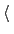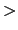Next: Extended Lagrangian. Up: Defining collective variables and Previous: Artificial boundary potentials (walls)   Contents   Index

## Trajectory output

• outputValueOutput a trajectory for this colvarContext: colvar
Acceptable values: boolean
Default value: on
Description: If colvarsTrajFrequency is non-zero, the value of this colvar is written to the trajectory file every colvarsTrajFrequency steps in the column labeledname''.

• outputVelocityOutput a velocity trajectory for this colvarContext: colvar
Acceptable values: boolean
Default value: off
Description: If colvarsTrajFrequency is defined, the finite-difference calculated velocity of this colvar are written to the trajectory file under the label v_name''.

• outputEnergyOutput an energy trajectory for this colvarContext: colvar
Acceptable values: boolean
Default value: off
Description: This option applies only to extended Lagrangian colvars. If colvarsTrajFrequency is defined, the kinetic energy of the extended degree and freedom and the potential energy of the restraining spring are are written to the trajectory file under the labels Ek_name'' and Ep_name''.

• outputTotalForceOutput a total force trajectory for this colvarContext: colvar
Acceptable values: boolean
Default value: off
Description: If colvarsTrajFrequency is defined, the total force on this colvar (i.e. the projection of all atomic total forces onto this colvar -- see equation (13.19) in section 13.5.1) are written to the trajectory file under the label fs_name''. For extended Lagrangian colvars, the total force'' felt by the extended degree of freedom is simply the force from the harmonic spring. Note: not all components support this option. The physical unit for this force is kcal/mol, divided by the colvar unit U.

• outputAppliedForceOutput an applied force trajectory for this colvarContext: colvar
Acceptable values: boolean
Default value: off
Description: If colvarsTrajFrequency is defined, the total force applied on this colvar by biases and confining potentials (walls) within the Colvars module are written to the trajectory under the label fa_name''. For extended Lagrangian colvars, this force is actually applied to the extended degree of freedom rather than the geometric colvar itself. The physical unit for this force is kcal/mol divided by the colvar unit.Next: Extended Lagrangian. Up: Defining collective variables and Previous: Artificial boundary potentials (walls)   Contents   Index
vmd@ks.uiuc.edu